# Grade - math word problems

#### Number of examples found: 5347

• TrianglesEquilateral triangle with side 40 cm has the same perimeter as an isosceles triangle with arm of 45 cm. Calculate the base x of an isosceles triangle.
• TrapezoidCalculate area of trapezoid ABCD with sides |AD|= 68 cm, |DC|=35 cm, |CB|=12 cm, |AB|=35 cm..
• What time is it?What time is it, when there is 4 times less time left until midnight than the time that has elapsed since noon? What time is it, when one-fifth of the hours that have passed since midnight is equal to one-third of the hours that are missing by 12 o'clock?
• Hemisphere cutCalculate the volume of the spherical layer that remains from the hemisphere after the 3 cm section is cut. The height of the hemisphere is 10 cm.
• FruitsIn the shop sell 4 kinds of fruits. How many ways can we buy three pieces of fruit?
• Four paversFour pavers would pave the square in 18 days. How many pavers do you need to add to done work in 12 days?
• Lake or pondThe landlord has a square lake. Trees grow around this lake. The lake wants to enlarge the pond twice and does not want to cut down or flood any tree. How will he do that?
• Cube surface and volumeFind the surface of the cube with a volume of 27 dm3.
• The sales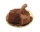The sales tax rate is 4.447​% for the city and 4​% for the state. Find the total amount paid for 2 boxes of chocolates at ​\$17.96 each.
• Father and sonFather and son weigh together 108kg. The father weighs 2.6 times more than his son. How much does weight my father and son count?
• Apples 14Maya bought 18 apples. She used 50% of the apples to make apple pies and 1/3 of the apples was mixed with other fruits to make salad. How many apples were left?
• A squareA square with length of diagonals 12cm give: a) Calculate the area of a square b) rhombus with the same area as the square, has one diagonal with length of 16 cm. Calculate the length of the other diagonal.
• Bridge cards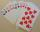How many bridge hands are possible containing 4 spades,6 diamonds, 1 club, and 2 hearts?
• Geometric seqFind the third member of geometric progression if a1 + a2 = 36 and a1 + a3 = 90. Calculate its quotient.
• Cuboid heightWhat is the height of the cuboid if the edges of its base are 15 cm and 4 cm long and its volume is 420 cm cubic?
• FriendsJames and Jaroslav are friends. Together they had 48 toy cars. But if John took 8 cars from Jaroslav, Jaroslav will be have 1.5 times more toy cars than James. How many toy cars had Jaroslav and James?
• Eight palm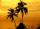There grows 8 palms by the sea. At the first sitting one parrot, on second two, on third sits four parrots on each other twice the previous parrots sitting on a previous palm. How many parrots sitting on eighth palm?
• TrapeziumThe area of trapezium is 35 cm2. Find its altitude if the bases are 6cm and 8 cm.
• Water colors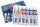Painting workshop attend 10 students. Eight students painted water colors and nine painted with tempera colors. How many students painted water colors and tempera at the same time?
• Two workers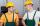Two workers work together and perform the job in 12 hours. If one has done half the work and then second worker next half of work it will take 25 hours. How long would it take job them separately?

Do you have an interesting mathematical word problem that you can't solve it? Submit a math problem, and we can try to solve it.

We will send a solution to your e-mail address. Solved examples are also published here. Please enter the e-mail correctly and check whether you don't have a full mailbox.

Please do not submit problems from current active competitions such as Mathematical Olympiad, correspondence seminars etc...